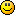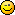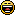# Just for fun

1. There's an obvious flaw in this. See who's first to spot it. Since the forum text doesn't support superscript, I'm using a^2 to mean a squared

if a = b, then:

a^2 = ab                (multiplying both sides by a)
a^2 - b^2 = ab - b^2    (subtracting b^2)
(a - b)(a + b) = b(a - b)   (factorising)
(a + b) = b             (dividing through by (a-b)
2b = b                 (because a=b)
2 = 1                  (dividing through by b)

1. Tough one - I was always crap at algebra. I would hazard a guess that a^2 - b^2 and ab - b^2 are both zero. Therefore the only result is 0 = 0 ?

1. Very close, but that's not quite the line that blows the whole thing up. Let's see if anyone else has a go, before I post the answer.

2. From the little mathematics I know ...

2 x 0 = 0
1 x 0 = 0

Therefore 2=1By the way, Paraglider, congratulations on your 300th fan ... any moment now ...1. I think your cut-down version is just too simple to fool anyone, but you never know!

Thanks for the fan-observation3. a^2 = ab                (multiplying both sides by a)
a^2 - b^2 = ab - b^2    (subtracting b^2)
(a - b)(a + b) = b(a - b)   (factorising)

(o)(2)= 1(0)

you are dividing 0 by 0

(a + b) = b             (dividing through by (a-b)
2b = b                 (because a=b)
2 = 1                  (dividing through by b)

4. Speak to me in quarter notes. I get that. This ain't no quarter note talk.
LOL

1. You being crotchety?2. This isn't fun, I have a hard time in Sudoku already3. My guess.

The step : "dividing through by (a-b)"

is invalid, because (a-b)=0 and x/0 = undefined

1. You're exactly right, because the whole premise is that a = b.

2. The correct answer = StefanMDP

3. And the prize goes to -

Exactly right. Division by zero is undefined (because the result tends towards infinity).

But you must admit it's a neat trick!

1. Darn it!! I was just coming in to answer the question well it certainly was a good one..4. No, no, no:  I see the obvious flaw here -- Paraglider, it's simple: there's no chocolate in it.  Just add chocolate for a perfect equation, every time.  Next?

1.0
pgrundyposted 14 years agoin reply to this

See, that's why you are my people.5.0
C. C. Riterposted 14 years ago

I don't see it: 2b=b

6. 2b, or not 2b? That's the real question.
Assuming a=b is the wrong assunption.7. Chocolate - sheeesh...

women . . .   (d&r)

8. Aww...congrats, Paraglidertis a time to celebrate. Will there be chocolate.... sighs

9. Oh! I missed out on ^. I was wondering what it was. Poor focus!

10. The Problem is in the Step 3, you are canceling out (a-b) both on the LHS as well as in the RHS. whereas a=b, and a-b=0, So divide by 0 error, as 0/0 is invalid operation ans will result in 0. As,
0/0= 0*(1/0). Anything multiplied by 0 is 0.

11. true - dividing by zero is still undefined, even after two months1.12. I like this sort of mental exercises, however I'm crap at maths:) Could you get any easier ones:)

13. I don't see Misha in this forum....
"(

working